Next: Carbon Seal Up: Fluid Section Types: Gases Previous: Straight and Stepped Labyrinth   Contents

### Characteristic

Properties: adiabatic, not isentropic, symmetric

Sometimes a network element is described by its characteristic curve, expressing the reduced mass flow as a function of the pressure ratio (Figure 91). This allows the user to define new elements not already available.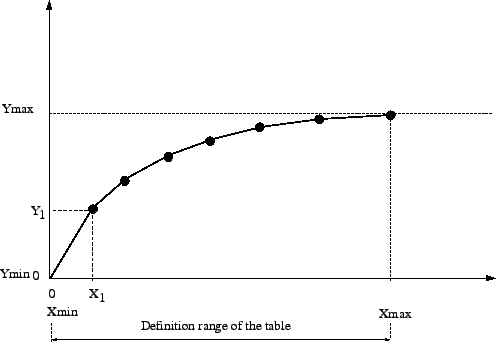The reduced flow is defined by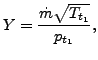(55)

where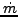is the mass flow,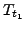is the upstream total temperature and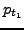is the upstream total pressure. Here upstream'' refers to the actual flow direction, the same applies to downstream''. The abscissa of the curve is defined by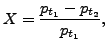(56)

where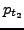is the downstream total pressure. Notice that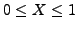. It is advisable to define Y for the complete X-range. If not, constant extrapolation applies. Notice that zero and small slopes of the curve can lead to convergence problems. This is quite natural, since the reduced flow corresponds to the left hand side of Equation(33), apart from a constant. Zero slope implies a maximum, which corresponds to sonic flow (cf. the discussion of Equation(33)). In general, some sort of saturation will occur for values of X close to 1.

The characteristic curve is defined by the following parameters (to be specified in that order on the line beneath the *FLUID SECTION, TYPE=CHARACTERISTIC card):

• scaling factor (default: 1)
• not used (internally: number of pairs)
• not used (internally: set to zero)
• not used (internally: set to zero)
•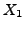•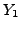•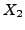•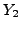Use more cards if more than two pairs are needed (maximum 16 entries per line, i.e. 8 pairs). No more than 10 pairs in total are allowed. In between the data points CalculiX performs an interpolation (solid line in Figure 91). In addition, the default point (0,0) is added as first point of the curve.

The scaling factor (first entry) is used to scale the ordinate values Y.

Example files: characteristic.Next: Carbon Seal Up: Fluid Section Types: Gases Previous: Straight and Stepped Labyrinth   Contents
guido dhondt 2018-12-15